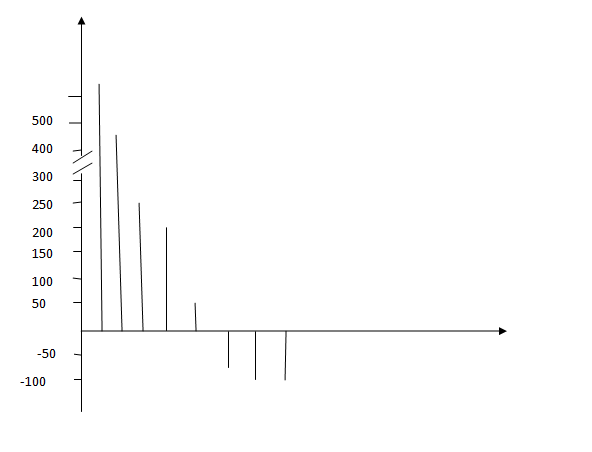## Uneven tick intervals with Linear Axis in GTL v9.3?

Is it possible to have evenly spaced ticks on a y-axis but at a certain point the underlying values between the ticks is increased?  For example, from 0 to 300 there is a tick mark every 50 but from 300 to 700 the tick marks remain at the same spacing but each now represent a difference of 100.  My statistician's mock-up is below to better visualize this.  I'm doing paneled needle plots using GTL in version 9.3.  Thanks!1 ACCEPTED SOLUTION

Accepted SolutionsJay54
Meteorite | Level 14

## Re: Uneven tick intervals with Linear Axis in GTL v9.3?

Yes, you can do this, however, I would be cautious.  While I defer to your statistician, this could provide the potential for misleading a reader of such a graph.   A user may not notice that the same tick interval represents a different amounts in the two parts.  In this blog article, I have presented some ways to handle this using GTL.

BTW:  With SAS

http://blogs.sas.com/content/graphicallyspeaking/2012/06/03/broken-y-axis-with-sas-9-2/

6 REPLIES 6Jay54
Meteorite | Level 14

## Re: Uneven tick intervals with Linear Axis in GTL v9.3?

Yes, you can do this, however, I would be cautious.  While I defer to your statistician, this could provide the potential for misleading a reader of such a graph.   A user may not notice that the same tick interval represents a different amounts in the two parts.  In this blog article, I have presented some ways to handle this using GTL.

BTW:  With SAS

http://blogs.sas.com/content/graphicallyspeaking/2012/06/03/broken-y-axis-with-sas-9-2/

## Re: Uneven tick intervals with Linear Axis in GTL v9.3?

Thanks for the help.  Believe me, I'm trying to talk her out of it but she still wants the data presented this way.  Below is what I have now and my new question is if I can control the scale of the tick marks?  What she's trying to do is reduce the space used in the upper portion of each plot so the lower portion has more space (in particular, the values of the x-axis on the right side from 0 to -100).  The example from your blog still has each tick representing a value of 5 despite the panel break but in this case she wants each tick in the upper plot to represent 100 and in the lower plot each tick to represent 50 but the ticks to be the same distance apart.  Is this possible?Jay54
Meteorite | Level 14

## Re: Uneven tick intervals with Linear Axis in GTL v9.3?

In my blog article, I intentionally made the tick interval the same.  You don't have to do that.  You can use any tick interval you want in each y axis.  If you don't set any y axis tick values, the axis will pick nice round numbers based on the data range of each Y axis.

## Re: Uneven tick intervals with Linear Axis in GTL v9.3?

I think I must not be asking my question clearly.  What I'm actually trying to do is make the top panel y-axis (the 300 to 600 section) "shorter" so that the bottom panel (-100 to 300) has more space and the needle bars will be longer.  Maybe it's not the tick marks that I'm concerned about but controlling the length of the axes.  My code is below and I've specified the tick marks as I want them but can I specify somehow that I want the axes to be different lengths?

proc template;
define statgraph wfpanel;

begingraph / designwidth=480 designheight=640;

layout lattice / rows=4 columns=1 columndatarange=union rowweights=(0.35 0.65) rowgutter=2;

columnaxes;
columnaxis / display=(line label) /*tickvalueattrs=(size=7)*/;
endcolumnaxes;

layout overlay / yaxisopts=(griddisplay=on display=(ticks tickvalues)
tickvalueattrs=(size=7)
offsetmin=0 linearopts=(viewmin=300 viewmax=600
tickvaluelist=(400 500 600)));
needleplot x=obs1 y=pchg_bst;
endlayout;
layout overlay / yaxisopts=(griddisplay=on display=(ticks tickvalues)
tickvalueattrs=(size=7)
offsetmin=0 linearopts=(viewmax=300 tickvaluelist=(-100 -50 0 50 100 150 200 250 300)));
needleplot x=obs1 y=pchg_bst;
endlayout;

layout overlay / yaxisopts=(griddisplay=on display=(ticks tickvalues)
tickvalueattrs=(size=7)
offsetmin=0 linearopts=(viewmin=300 viewmax=600
tickvaluelist=(400 500 600)));
needleplot x=obs2 y=pchg_bst;
endlayout;
layout overlay / yaxisopts=(griddisplay=on display=(ticks tickvalues)
tickvalueattrs=(size=7)
offsetmin=0 linearopts=(viewmax=300 tickvaluelist=(-100 -50 0 50 100 150 200 250 300)));
needleplot x=obs2 y=pchg_bst;
endlayout;

endlayout;

endgraph;

end;

run;Jay54
Meteorite | Level 14

## Re: Uneven tick intervals with Linear Axis in GTL v9.3?

You cannot specify the "Length" of each axis (in pixels on the screen).  The axis length is determined by the height of each cell.  You can use ROWWEIGHTS on the LAYOUT LATTICE to specify the proportional height of each cell.  Default is equal weights for all rows.

The data range represented on each axis is the union of all the data ranges from all the plots in the cell.  You can specify to see less or more data range mapped to each axis by using the VIEWMIN and VIEWMAX options in the LINEAROPTS bundle on the YAXISOPTS.  You can also set the tick values you want to see.  If you don't set viewmin / viewmax or values, you will get the default behavior.  Note in the program above, ROWWEIGHTS are set and also the Y axis viewmin, viewmax and values.

## Re: Uneven tick intervals with Linear Axis in GTL v9.3?

Yes!  Rowweights...that's what I needed.  Thanks so much!

Discussion stats
• 6 replies
• 2356 views
• 3 likes
• 2 in conversation